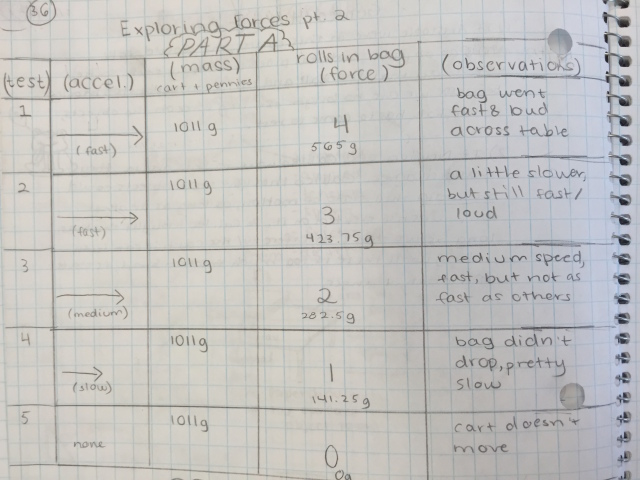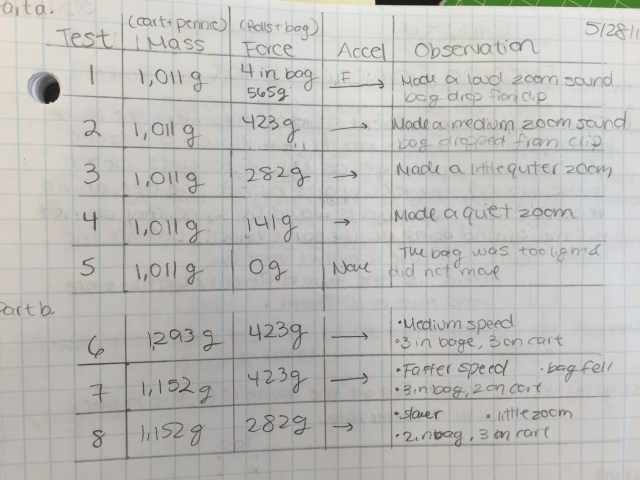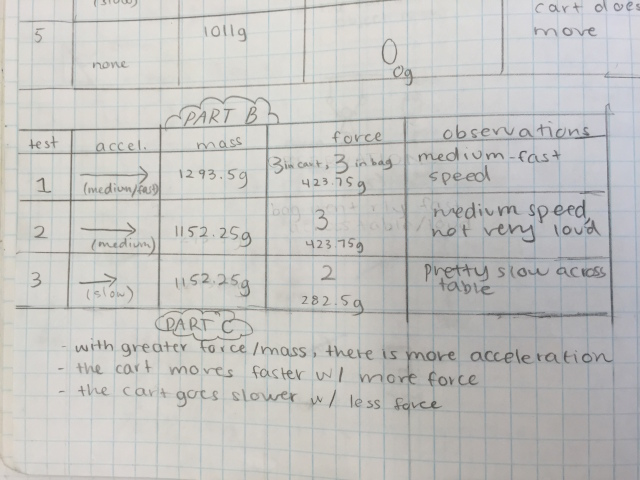# Exploring Forces (Part 2)

19 teachers like this lesson
Print Lesson

## Objective

SWBAT use a model to understand the relation between force, mass, and acceleration.

#### Big Idea

Use this lesson to help students make sense of Newton's Second Law.

## Getting Started

Materials needed per group of students:

• Cart
• Rolls of pennies (4 or more)
• Sandwich bags
• Medium size binder clip
• String

## Engage

5 minutes

Begin by asking students to think about following scenario

"Suppose you know how hard you have to push to get a small box to accelerate a certain amount. If you want a heavier box to accelerate at the same rate, what do you have to do? If you want the small box to accelerate at a greater rate, what do you have to do?"

Give students a moment to think and then ask for their ideas.

## Explore

25 minutes

Begin by asking students to predict what they think will happen to acceleration as the applied force changes in each trial.

For instance, thinking about the Exploring Forces Part 2 investigation, they might predict that when the applied force (pennies in the bag) is greatest (4 penny rolls), the acceleration will be greatest.

As the applied force lessens (3, 2, or 1 penny roll(s)) acceleration will be less. Since the mass is the measurement of the entire system, it will be be equal to the mass of the cart (4.4 N) plus the mass of the four penny rolls (5.6 N) or 10N.

If they take penny rolls away from the system (using only three for instance), the relationship between force  and acceleration will not change. If we are considering mass as the total mass of the system, then it will be the calculation of the total available applied force plus the mass of the cart.

For the investigation. students assemble the materials and perform the investigation as described. Starting with four penny rolls in the bag and zero on the cart (0/4), they suspend the bag just off the edge of the table and let it go. The cart should accelerate very quickly and roll off of the table.

Next, they remove one roll of pennies from the bag and place them on the cart (1/3), release the bag and observe the cart accelerate at a rate somewhat slower than the previous trial.

Repeat this for 2/2, 3/1 and 4/0. The acceleration decreases as the applied force of the pennies decreases. In the last trial, there is no acceleration since there is no applied force acting on the cart.

Since students tend to struggle with the use of the terms mass and weight, I have included an article titled "The Great Mass Weight Confusion" that you can hand out to students and have them read to clarify the scientific underpinnings of these terms.

As students report their results the pattern should be fairly obvious, acceleration of the cart is greatest when the force applied is the greatest. As the applied force decreases, so does the acceleration. The mass is constant throughout all trials (make sure they don't overlook this).## Explain

15 minutes

Since we were to thinking of mass as the mass of the entire system, this number is constant for the first five trials (10N). If you manipulate the system by removing penny rolls, the total mass of the system is still the calculation of the total penny rolls used plus the cart.

Force and acceleration changes depending on these configurations. More applied force (penny rolls in the bag) means greater acceleration. As the applied force decreases, the rate of acceleration decreases.

Whenever you change the force, the acceleration changes as well. If you double, triple or quadruple the force, the acceleration increases accordingly.In the video below  I briefly discuss how this lesson aligns with the science practice of analyzing and interpreting data.

## Elaborate

15 minutes

To get a closer look at the relationships of force, mass and acceleration, have students take a look at the videos that show different distributions of penny rolls. In these videos, I've always used four penny rolls and distributed them between the cart and the bag so that the total mass of the system is always the same - the cart plus four penny rolls.

Before they watch the videos, ask them to predict what will happen.Have them draw a diagram in which they label the mass, the force, and the acceleration.

To distinguish between force and acceleration in the diagram, they may want to use different colors or arrows of different thickness.

The focus is on how much acceleration (qualitatively, compared to the other videos)  they think each combination of mass and force will produce? In which scenarios will the magnitude of the acceleration be larger?

## Extend

15 minutes

After your students have experimented with the cart and penny roll systems, where the mass stays the same, try these variations:

1. Add more mass to the cart (whatever you can fit in), but keep the force (the number of hanging penny rolls) the same. What do you observe? F = same, m = smaller, acceleration = ?
2. Take a penny roll or two out of the cart but keep the force (the hanging penny rolls) the same. What do you observe? F = same, m = smaller, acceleration = ?

Ask them to report their predictions, procedures, results, and explanations.

1. What questions emerge?

2. What is your understanding of the relationships among force, mass, and acceleration?

As they continue to discuss their findings, have them examine the evidence that they have accumulated.

1. Are there patterns? Are there discrepancies?
2. What is their understanding of the formula F=ma, Newton's second law of motion?